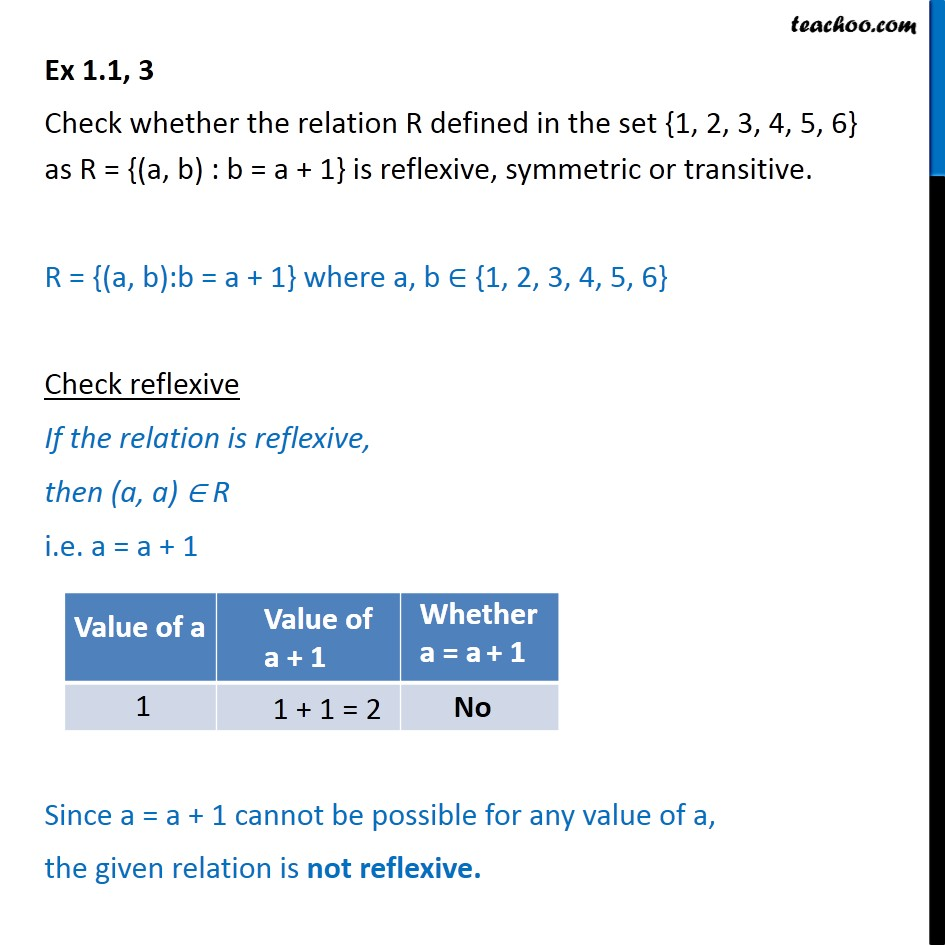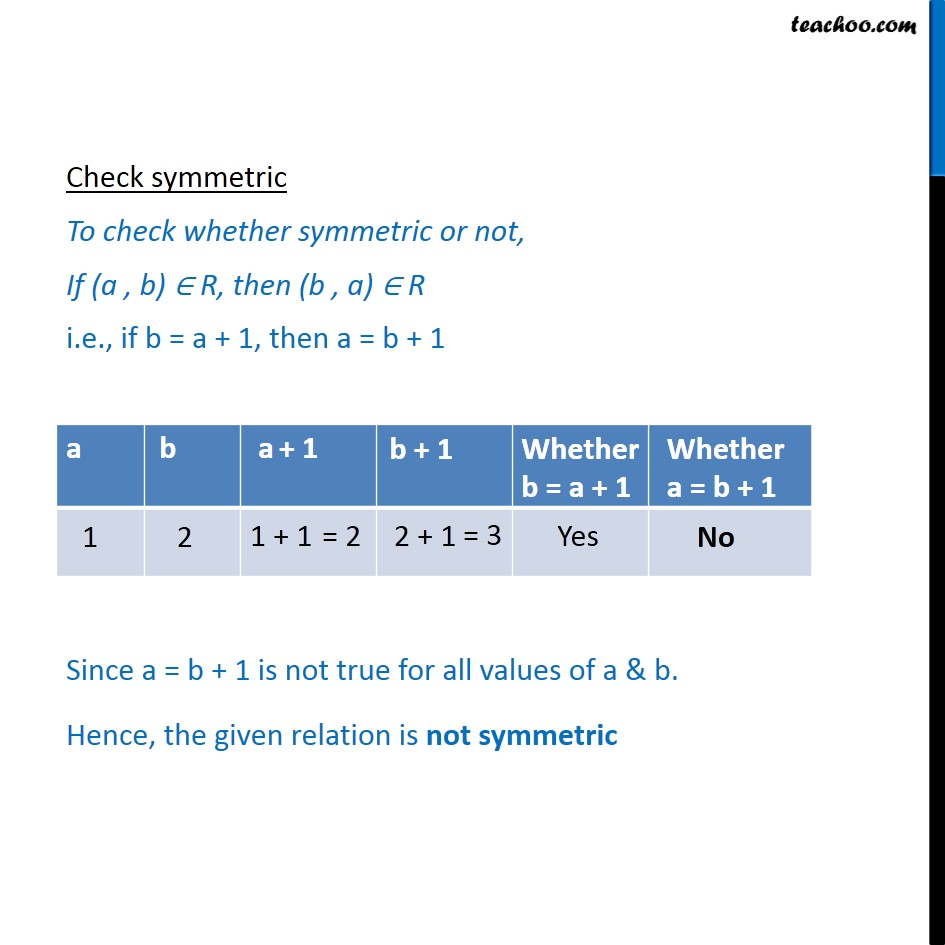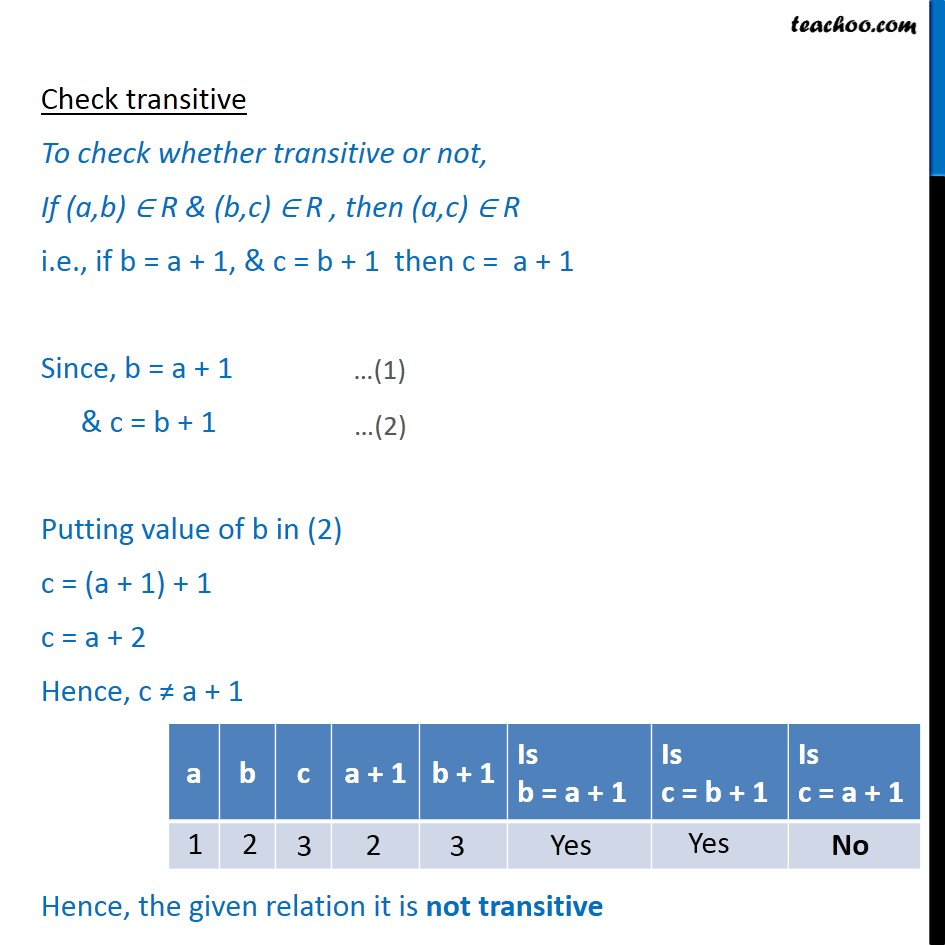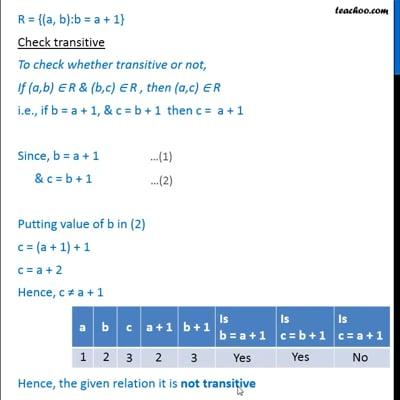Ex 1.1

Chapter 1 Class 12 Relation and Functions
Serial order wiseThis video is only available for Teachoo black users

Introducing your new favourite teacher - Teachoo Black, at only ₹83 per month

### Transcript

Ex 1.1, 3 Check whether the relation R defined in the set {1, 2, 3, 4, 5, 6} as R = {(a, b) : b = a + 1} is reflexive, symmetric or transitive. R = {(a, b):b = a + 1} where a, b {1, 2, 3, 4, 5, 6} Check reflexive If the relation is reflexive, then (a, a) R i.e. a = a + 1 Since a = a + 1 cannot be possible for any value of a, the given relation is not reflexive. Check symmetric To check whether symmetric or not, If (a , b) R, then (b , a) R i.e., if b = a + 1, then a = b + 1 Since a = b + 1 is not true for all values of a & b. Hence, the given relation is not symmetric Check transitive To check whether transitive or not, If (a,b) R & (b,c) R , then (a,c) R i.e., if b = a + 1, & c = b + 1 then c = a + 1 Since, b = a + 1 & c = b + 1 Putting value of b in (2) c = (a + 1) + 1 c = a + 2 Hence, c a + 1 Hence, the given relation it is not transitive